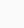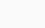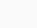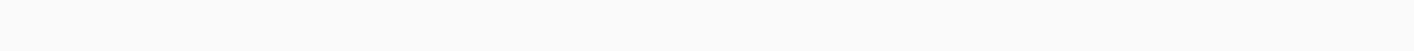msomi maktaba

# Form 4 Mathematics – LINEAR PROGRAMMING

SIMULTANEOUS EQUATIONS
Solving simultaneous equations graphically, the solution is given by the point of intersection of the two lines.

Examples

1.  Solve graphically the following simultaneous equations.

2x – y = 1

3x+3y =6

Equation 1
2x – y = 1

X intercept, y= 0

2x – y = 1

2x – 0 = 1

x =(, 0)

Y  intercept, x = o

2x – y = 1

y = 1

(0,  -1)

Equation  2.

3x + 3y = 6

X intercept,Y=0

3x-0  =  6

X = 2

(2,0)

Y intercept,X=0

0 + 3y = 6

3Y = 6

y = 2

(0,2)Solution ( 1,1) i.e x = 1 and y = 1.

2.      Ali paid 34 shillings for 10 oranges and 35 mangoes. Moshi went to the same fruit market and paid 24 shillings for 16 oranges and 18 mangoes . what was the price of a mango and for an orange.

Solution:

Let X be a price of an orange

Let y be the price of mangoes

10X + 35 Y = 34

16X + 18 Y = 24

Solution:380 y = 314

Y = 0.8

10X + 35y = 34

10X + 35 x 0.8 = 34

10X + 28 = 34

X= 0.6

X intercept,Y=0

10 X + 35 Y = 34

10X + 0 = 34

10 X = 34

X = 3.4                 (3.4,0)

Y intercept , X=0

0 + 35 Y = 34

35Y = 34

Y = 1                  (0,1)

X intercept Y =0

16X + 18 Y = 24

16X = 24

X = 1.5                 (1.5, 0)

Y intercept X= 0

18Y = 24

Y = 1.3*               (0 ,1.3)x = 0.6
y = 0.8
...Price of an orange is 0.6sh and price of a mango is 0.8sh.

3.      7 X + 3Y + 12 = 0

5X – 2Y + 2 = 0

Solution:

7X + 3Y + 12 = 0

X intercept y=0

7X + 0 = -12

X =Y intercept X=0

0 + 3Y = -12

3Y = -12

Y = 4

5X – 2Y = -2

X intercept  y=0

5X- 0 = -2

X=Y intercept X= 0

0 – 2y = -2

Y = 1

4.      2c + 2d  = c – d

2d + 2 = c + 1

Solution:

2c + 2d = c – d

2c –c + 2d + d = 0

c + 3d =0…….(1)

2d + 2 = c + 1

2d + 2- c-1= 0

2d – c + 1= 0 ……..(2)

c + 3d = 0

c intercept, d = 0

c = 0

d intercept , c = 0

3d  = 0

d = 0

2d – c = -1

C intercept, d= 0

-c = -1

c = 1

d intercept , c= 0

0 – 2d = 1

d = –d = (0, – 0.5)Solution (0.6, -0.2)

By substitution method

c + 3d = 0 ——-(i)

c – 2d = 1——–(ii)

c = -3d

c -2d = 1

c – 2(-0.2) = 1

c + 0.4 = 1 – 0.4

c = 0.6

:.  c = 0.6 , d = -0.2

LINEAR INEQUALITIES

When  a linear equation of the form ax + by + c = 0 is represented on a coordinate plane, it separates the plane into two disjoint sets.
E.g. y = 4 separate the plane into two disjoint sets
The points above the line y= 4 satisfy the relation y>4, while those in the lower half plane satisfy the relation y < 4.

When drawing the inequality y > 4, first draw the line of separation to separate the plane y>4, and the plane y< 4 which is y = 0, Then shade the unwanted region.Note: The shaded region together with the line y = 4 represents y > 4

Boundaries of half- plane
Equations are used to describe the boundaries of half- plane. The boundary lines are continuous to include points on the line when the inequality is written using the sign > or < where as the boundary lines are dotted if the inequality is written in the of form < or >.
E.g. Draw and show the half- plane representing the inequality 4x + 2y > 8Feasible Region
Feasible region is the region of intersection of all the inequality given in a problem. In order the feasible region to be seen clearly the unwanted region of the inequalities should be shaded leaving the required region clear.
Example 1.
Show the feasible region which satisfy the following inequalities 3x + 3y > 12 and y – x < 2
Solution
3x + 3y > 12,      (0, 4) (4, 0)
y – x < 2,             (0, 2) (-2, 0)From 3x + 3y > 12, after drawing the line of separation i.e 3x + 3y = 12, The point above or below the line is used to test which region satisfy the inequality.
e.g. (0, 0) the origin
3(0) + 3(0) > 12
0> 12 Not true
... The region below the line does not satisfy the inequality instead. The opposite region satisfies.The region below is shaded to live the wanted region clear.

For the case of y – x < 2
testing using the origin (0, 0)
0 – 0 < 2
0 < 2 (it is true)
The region below is the wanted region, therefore we shade the region above.

Example 2

Solution:

Let Y be the no of Royal bread

200X + 250Y ≤ 36000

X + Y ≤ 150

X ≥ 0

Y ≥ 0

X intercept, Y=0

200X+ 250Y = 36,000

200X = 36000

X = 180

(180,0)

X intercept, Y = 0

X + Y = 150

X = 150

(150, 0)

Y intercept, X = 0

0 + 250 Y = 36,000

250Y = 36,000

Y = 144

( 0, 144)

Y intercept, X = 0

0 + Y = 150

Y = 150

objective function

40X + 50/100 x 250Y = Maximum profit

40X + 125 Y = Maximum profit

A (6, 0)

B (144, 0)

C (0,144)

40X + 125Y = Maximum profit

40 + 125X144 =18,040The Corner points
A (0, 0)                      C (30, 120)
B (0, 144)                  D  (150, 0)

f(x, y) = 40x + 125y maximize

f(0, 0)       = 40x + 125y  = 0
f(0, 144)   = 40x + 125y   = 18000
f(30, 144) = 40x + 125y   = 15120
f(150, 0)   = 40x + 125y   = 6000

optimal point is (0, 144)
... In order for the bread dealer to get maximum profit, he should prepare 144 royal breads only.

Example 3.
Draw a graph and show the feasible region which is satisfied by the inequalities ;

X ≥ 0,  2x + y ≤ 4,   2x +3y ≥ 8  , y ≥ 0 , y+ 3x = 9 , x + y ≤ 10

Which If any of the inequalities can be omitted without affecting the answer.

SOLUTION

2x + y = 4

X intercept, Y = 0

2x + 0 =4

x = 2

(2, 0)

Y intercept

0 + y = 4

y = 4

(0, 4)

2x + 3y = 8

X intercept, Y =0

2x + 0 = 8

2x = 8

x =4

(4, 0)

y intercept, x =0

0 + 3Y = 8

y =8/3

(0 , 2.7)

x + 3y = 9

x intercept, y =0

x + 0 = 9

x = 9

y intercept, y = 0

0 + 3Y = 9

y = 3

(0, 3)

x + y = 10

x intercept, y =0

x =10

(10, 0)

y intercept, x =0

y = 10

(0, 10 )Example 4.
George buys X pencils 10 shillings each,( X+8) exercise books at 10 shillings each. If he wishes to have some change as town bus fare from a 200shs note, form an inequality in X and solve it to find the range of X.

Solution:

X no. of pencils

0 X + 10(X+8) ≤200

10X + 10X + 80 = 200

20 X = 120

X = 6

X < 6

The range of x is = 1, 2,3,4,5.

Exercise 8.1
1. Show by shading out the unwanted regions, the half planes representing the following simultaneous inequalities.
a) y < 2x – 1, y > 3 – x
b) -3 < x – y<2
c) y< 2x, y > 3 – x, y > -1

Solution

a)   y < 2x – 1                                                      y > 3 – x
1 < 2x – y                                                      y + x > 3
2x – y> 1                                                       y + x = 3
2x – y = 1                                                     (0, 3) (3,0)
(0, -1) (1/2, 0)(b) -3 < x – y < 2
-3 < x – y                                                         x – y < 2
x – y > -3                                                         x – y = 2
x – y = -3                                                           (0, -2) ( 2, 0)
(0, 3) (-3, 0)(c) y > 2x – 1                                                     y > 3 – x
y – 2x > -1                                                    y + x > 3
y – 2x = -1                                                    y + x = 3
(0, -1) (1/2, 0)                                              (0, 3) (3, 0)
y > -1
y = -1Linear Programming
Linear programming is a branch of mathematics which enables to solve problems which one wants to get the greatest or least value of a quantity.

Solving linear programming problems
1.A farm is to be planted with wheat and maize while observing the following.If wheat yields a profit of 800 Sh. per hectare while maiza yields 600sh.per hectare.How should the area be planted in order to get maximum profit?

Solution
Step1:
Express the information provided in mathematical form
Let x represents number of hectares of wheat planted
y represents number of hectares of maize to be planted

Step2: The objective function
This is the function to be optimized. The objective of the problem is to maximize the profit. Therefore
800x – profit yielded by wheat
600y – profit yielded by maize
The objective function f(x,y) = 800x + 600y is to be maximized.

Step3.Availability.
2x + y < 10 (Available days of labor)
700x + 600y < 4200 (Available money for labors cost)
300x + 400y < 2400 (Available money for fertilizer)
x > 0, y>0 hectares of wheat and maize can only be positive. (non – negative)
... The problem is to maximize f(x,y) = 800x + 600y subject to
2x + y < 10
700x + 600y < 4200   i.e    7x + 6y < 42
300x + 400y < 2400   i.e    3x + 4y < 24
x > 0,
y>0
Step4.Plot the constraints on the graph
2x + y < 10      (0,10 ) (5,0 )
7x + 6y < 42     (0,7 ) (6,0 )
3x + 4y < 24     (0,6 ) (8, 0)step 5.The Identify the corner points.
A(0, 6), B(0,0), C(5,0), D(3.3, 2.4), E(2.4, 4.2)

Step6.Substitute the corner points to the objects function.
f(x, y)       = 800x + 600y
f(0, 6)       = 800x + 600y  = 3600
f(0, 0)       = 800x + 600y  = 0
f(5, 0)       = 800x + 600y  = 40000
f(3.8, 2.4) = 800x + 600y  = 4480
f(2.4, 4.2) = 800x + 600y  = 4500

Note: The point gives the best solution is called optimal point and the best solution is called the optimal solution
... f(2.4, 4.2) is the optimal point and 4500 is the optimal solution.
2.4 hectares should be planted wheat and while 4.2 hectares should be planted maize in order to get maximum profit

EXERCISE 8.2

1. A doctor prescribes that in order obtain an adequate supply of Vitamins A and C, his patient shall have portions of food 1 and 2. The number of the units of vitamin A and C are given in a table below,The doctor prescribes a minimum of 14 units of vitamin A and 21 units of vitamin C. What are the least number of portion of food 1 and 2 that will fit the doctors prescription?

Solution
Let x represents number of portion of food 1 and y represent number of portion of food 2.3x + y > 14
2x + 7y > 21
x > 0
y > 0
Objective function
To minimize f(x,y) = x + y
To represents the constraint on the x-y plane
3x + y = 14      (0, 14) (4.667, 0)
2x +7y = 21     (0, 3) (10.5, 0)
x = 0
y = 0Corner points
A(0, 14)  B(4.03, 1.9)  C(10.5, 0)
f(x,y)          = x + y
f(0, 14)       = 0 + 14
f(4.03, 1.9) = 4.03 + 1.9
f(10.5, 0)    = 10.5 + 0

Best solution(optimal solution) = 5.93 and the optimal point is (4.03, 1.9).
... 4.03 is the portion of the food 1 and 1.9 is the portion of food 2 that should be taken to fit the doctors prescription.This site uses Akismet to reduce spam. Learn how your comment data is processed.